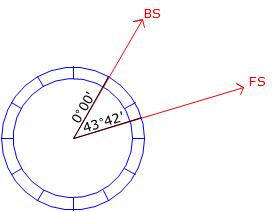## 1. Before We Start...

### a. Angle Sets

Angles are generally measured as direct and reverse (D/R) sets. How many sets are needed and how close the direct and reverse angles must be to each other depends on a number of things: survey purpose, TSI resolution, sight lengths, etc. 2. Incrementing the Circle(a) First measurement(b) Second measurement(c) Third measurement Figure C-1 Advancing the Circle